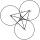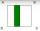Triangle SAA

The triangle has one side long 71 m and its two internal angles is 60°. Calculate the perimeter and area of the triangle.

Result

p =  213 m
A =  2182.82 m2

Solution:Try calculation via our triangle calculator.

Leave us a comment of example and its solution (i.e. if it is still somewhat unclear...):Be the first to comment!Next similar examples:

1. Three sidesSide b is 2 cm longer than side c, side a is 9 cm shorter than side b. The triangle circumference is 40 cm. Find the length of sides a, b, c . .. .
2. Height 2Calculate the height of the equilateral triangle with side 38.
3. SAS triangleThe triangle has two sides long 7 and 19 and included angle 36°. Calculate area of this triangle.
4. TriangleCalculate heights of the triangle ABC if sides of the triangle are a=75, b=84 and c=33.
5. The farmerThe farmer would like to first seed his small field. The required amount depends on the seed area. Field has a triangular shape. The farmer had fenced field, so he knows the lengths of the sides: 119, 111 and 90 meters. Find a suitable way to determine th
6. Isosceles IIIThe base of the isosceles triangle is 17 cm area 416 cm2. Calculate the perimeter of this triangle.
7. SinesIn ▵ ABC, if sin(α)=0.5 and sin(β)=0.6 calculate sin(γ)
8. Equilateral triangle v2Equilateral triangle has a perimeter 36 dm. What is its area?
9. CirclesThree circles of radius 95 cm 78 cm and 64 cm is mutually tangent. What is the perimeter of the triangle whose vertices are centers of the circles?
10. Center traverseIt is true that the middle traverse bisects the triangle?
11. Circular lawnAround a circular lawn area is 2 m wide sidewalk. The outer edge of the sidewalk is curb whose width is 2 m. Curbstone and the inner side of the sidewalk together form a concentric circles. Calculate the area of the circular lawn and the result round to 1
12. AceThe length of segment AB is 24 cm and the point M and N divided it into thirds. Calculate the circumference and area of this shape.
13. Folded squareABCD is a square. The square is folded on the midpoint of AB and A is folded onto the fold, creating a shaded region. The perimiter of the shaded figure is 75. Find the area of square ABCD
14. TrigonometryIs true equality? ?
15. Theorem proveWe want to prove the sentence: If the natural number n is divisible by six, then n is divisible by three. From what assumption we started?
16. Nineteenth memberFind the nineteenth member of the arithmetic sequence: a1=33 d=5 find a19
17. First manWhat is the likelihood of a random event where are five men and seven women first will leave the man?